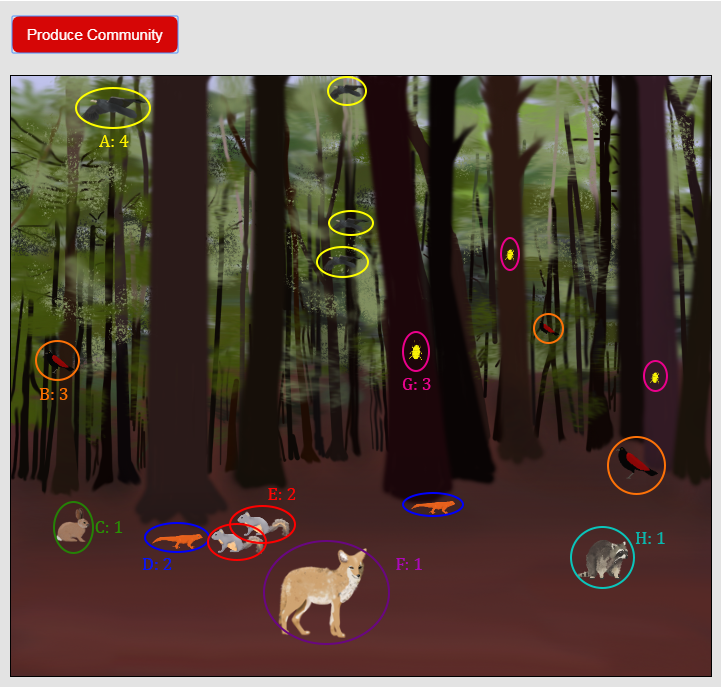Search
•Jolene Pappas

# How to calculate Simpson's Diversity Index (AP Biology)

Updated: Nov 20, 2020

Simpson's Diversity Index (SDI) is one approach to quantifying biodiversity. There are a number of other options that may be used (such as species richness and Shannon's Diversity Index), but the AP Biology Equation and Formula Sheet includes Simpson's, so AP Biology students should be prepared to use it for the AP Biology exam. SDI takes both the number of species and the population size of each species into account. The resulting value is between 0 and 1, with 0 representing no diversity (all individuals in an area are the same species) and 1 representing maximum diversity.

This post uses the version of SDI found on the AP Biology formula sheet. Another version of the equation is used for small communities. The two versions are sometimes called finite (small samples) and infinite (large samples). AP Biology uses the infinite version of the equation. The specific formula that appears on the AP exam will be used here, even though the simulations used for these examples produce small sample sizes. The Resources page (and Google drive) includes worksheets for both versions of SDI as well as species richness options.Two versions of Simpson's Diversity Index

Quick ecology vocab review before getting into the equation; a community is a group of different species in a given area and a population is a group of individuals of the same species in an area.

Two variables are needed for this formula. First is the total number of individuals in the community. Second is the population size for each species. In a real study, scientists use various sampling techniques to estimate population sizes. For the purposes of practice, we will use a simulation to collect data.Simpson's Diversity Index (infinite)

There are two simulations on Biology Simulations that lend themselves well to practicing diversity calculations. For our first example, we'll use the Biodiversity simulation. In this simulation, each time the "Produce Community" button is clicked, an animal community is produced in the forest ecosystem. Random components include the total number of individuals and the number of species represented. Pictured below is a sample run of the simulation, with each species circled in a different color. Identifying each species by name isn't important to completing the calculation, you just need to keep track of how many individuals are in each population.Animal Community

The table below displays the results for the sample run.Sample data from Biodiversity simulation

The total number of animals recorded (N) is 17. For the SDI calculation, we divide each of the species numbers (n) by the total (N) and then square the resulting value. Once that is complete for each species, add all those values together (that's what the summation symbol --capital sigma-- indicates). The final step is to subtract the resulting number from 1. The image below shows the calculation for the sample data.SDI sample calculation

For this sample data, Simpson's Diversity Index is 0.846. This value can be used to compare different ecosystems or examine change over time within an ecosystem. Next, we'll run a second example using the Macroinvertebrate simulation. Each time "Click here to collect macroinvertebrates" is clicked, a new sampling of organisms is produced. For this example, we'll run the simulation ten times to collect more data.Results from the Macroinvertebrate simulation

In the ten simulation runs a total of 65 individuals were "collected". This is still pretty small for using the infinite population version of the equation but again will work for practice purposes. The cumulative data is in the table below.Sample data from Macroinvertebrate simulation

Calculations for the second example are shown below.SDI sample calculation

The diversity index for the second example is 0.856. Based on these results, the forest ecosystem from the first example (0.846) is a little less diverse than the river ecosystem in the second example (0.856).

While decisions about sampling and quantifying biodiversity for actual ecosystems can be complex, calculating Simpson's Diversity Index based on simple population data is relatively straightforward.